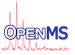OpenMS
OpenSwath_Scores Struct Reference

A structure to hold the different scores computed by OpenSWATH. More...

#include <OpenMS/ANALYSIS/OPENSWATH/OpenSwathScores.h>

Collaboration diagram for OpenSwath_Scores:
[legend]

## Public Member Functions

OpenSwath_Scores ()=default

double get_quick_lda_score (double library_corr_, double library_norm_manhattan_, double norm_rt_score_, double xcorr_coelution_score_, double xcorr_shape_score_, double log_sn_score_) const

double calculate_lda_prescore (const OpenSwath_Scores &scores) const
A quick LDA model based non-DIA scores. More...

double calculate_lda_single_transition (const OpenSwath_Scores &scores) const
A scoring model for peak groups with a single transition. More...

double calculate_swath_lda_prescore (const OpenSwath_Scores &scores) const
A full LDA model using DIA and non-DIA scores. More...

## Public Attributes

double elution_model_fit_score = 0

double library_corr = 0

double library_norm_manhattan = 0

double library_rootmeansquare = 0

double library_sangle = 0

double norm_rt_score = 0

double isotope_correlation = 0

double isotope_overlap = 0

double massdev_score = 0

double xcorr_coelution_score = 0

double xcorr_shape_score = 0

double yseries_score = 0

double bseries_score = 0

double log_sn_score = 0

double weighted_coelution_score = 0

double weighted_xcorr_shape = 0

double weighted_massdev_score = 0

double ms1_xcorr_coelution_score = -1

double ms1_xcorr_coelution_contrast_score = 0

double ms1_xcorr_coelution_combined_score = 0

double ms1_xcorr_shape_score = -1

double ms1_xcorr_shape_contrast_score = 0

double ms1_xcorr_shape_combined_score = 0

double ms1_ppm_score = 0

double ms1_isotope_correlation = 0

double ms1_isotope_overlap = 0

double ms1_mi_score = -1

double ms1_mi_contrast_score = 0

double ms1_mi_combined_score = 0

double sonar_sn = 0

double sonar_diff = 0

double sonar_trend = 0

double sonar_rsq = 0

double sonar_shape = 0

double sonar_lag = 0

double im_xcorr_coelution_score = 0

double im_xcorr_shape_score = 0

double im_delta_score = 0

double im_ms1_delta_score = 0

double im_drift = 0

double im_drift_weighted = 0

double im_delta = -1

double im_ms1_contrast_coelution = 0

double im_ms1_contrast_shape = 0

double im_ms1_sum_contrast_coelution = 0

double im_ms1_sum_contrast_shape = 0

double im_ms1_drift = 0

double im_ms1_delta = -1

double library_manhattan = 0

double library_dotprod = 0

double intensity = 0

double total_xic = 0

double nr_peaks = 0

double sn_ratio = 0

double mi_score = 0

double weighted_mi_score = 0

double rt_difference = 0

double normalized_experimental_rt = 0

double raw_rt_score = 0

double dotprod_score_dia = 0

double manhatt_score_dia = 0

## Detailed Description

A structure to hold the different scores computed by OpenSWATH.

This struct is used to store the individual OpenSWATH (sub-)scores. It also allows to compute some preliminary quality score for a feature by using a predefined combination of the individual scores determined using LDA.

## ◆ OpenSwath_Scores()

 OpenSwath_Scores ( )
default

## ◆ calculate_lda_prescore()

 double calculate_lda_prescore ( const OpenSwath_Scores & scores ) const

A quick LDA model based non-DIA scores.

A quick model LDA average model on which uses only non-DIA scores (library_corr, library_norm_manhattan, norm_rt_score, xcorr_coelution_score, xcorr_shape_score, log_sn_score and elution_model_fit_score).

Returns
A score which is better when more negative

## ◆ calculate_lda_single_transition()

 double calculate_lda_single_transition ( const OpenSwath_Scores & scores ) const

A scoring model for peak groups with a single transition.

Manually derived scoring model for single transition peakgroups, only uses norm_rt_score, log_sn_score, and elution_model_fit_score.

Returns
A score which is better when more negative

## ◆ calculate_swath_lda_prescore()

 double calculate_swath_lda_prescore ( const OpenSwath_Scores & scores ) const

A full LDA model using DIA and non-DIA scores.

A LDA average model which uses all available scores.

Returns
A score which is better when more negative

## ◆ get_quick_lda_score()

 double get_quick_lda_score ( double library_corr_, double library_norm_manhattan_, double norm_rt_score_, double xcorr_coelution_score_, double xcorr_shape_score_, double log_sn_score_ ) const

## ◆ bseries_score

 double bseries_score = 0

## ◆ dotprod_score_dia

 double dotprod_score_dia = 0

## ◆ elution_model_fit_score

 double elution_model_fit_score = 0

## ◆ im_delta

 double im_delta = -1

## ◆ im_delta_score

 double im_delta_score = 0

## ◆ im_drift

 double im_drift = 0

## ◆ im_drift_weighted

 double im_drift_weighted = 0

## ◆ im_ms1_contrast_coelution

 double im_ms1_contrast_coelution = 0

## ◆ im_ms1_contrast_shape

 double im_ms1_contrast_shape = 0

## ◆ im_ms1_delta

 double im_ms1_delta = -1

## ◆ im_ms1_delta_score

 double im_ms1_delta_score = 0

## ◆ im_ms1_drift

 double im_ms1_drift = 0

## ◆ im_ms1_sum_contrast_coelution

 double im_ms1_sum_contrast_coelution = 0

## ◆ im_ms1_sum_contrast_shape

 double im_ms1_sum_contrast_shape = 0

## ◆ im_xcorr_coelution_score

 double im_xcorr_coelution_score = 0

## ◆ im_xcorr_shape_score

 double im_xcorr_shape_score = 0

## ◆ intensity

 double intensity = 0

## ◆ isotope_correlation

 double isotope_correlation = 0

## ◆ isotope_overlap

 double isotope_overlap = 0

## ◆ library_corr

 double library_corr = 0

## ◆ library_dotprod

 double library_dotprod = 0

## ◆ library_manhattan

 double library_manhattan = 0

## ◆ library_norm_manhattan

 double library_norm_manhattan = 0

## ◆ library_rootmeansquare

 double library_rootmeansquare = 0

## ◆ library_sangle

 double library_sangle = 0

## ◆ log_sn_score

 double log_sn_score = 0

## ◆ manhatt_score_dia

 double manhatt_score_dia = 0

## ◆ massdev_score

 double massdev_score = 0

## ◆ mi_score

 double mi_score = 0

## ◆ ms1_isotope_correlation

 double ms1_isotope_correlation = 0

## ◆ ms1_isotope_overlap

 double ms1_isotope_overlap = 0

## ◆ ms1_mi_combined_score

 double ms1_mi_combined_score = 0

## ◆ ms1_mi_contrast_score

 double ms1_mi_contrast_score = 0

## ◆ ms1_mi_score

 double ms1_mi_score = -1

## ◆ ms1_ppm_score

 double ms1_ppm_score = 0

## ◆ ms1_xcorr_coelution_combined_score

 double ms1_xcorr_coelution_combined_score = 0

## ◆ ms1_xcorr_coelution_contrast_score

 double ms1_xcorr_coelution_contrast_score = 0

## ◆ ms1_xcorr_coelution_score

 double ms1_xcorr_coelution_score = -1

## ◆ ms1_xcorr_shape_combined_score

 double ms1_xcorr_shape_combined_score = 0

## ◆ ms1_xcorr_shape_contrast_score

 double ms1_xcorr_shape_contrast_score = 0

## ◆ ms1_xcorr_shape_score

 double ms1_xcorr_shape_score = -1

## ◆ norm_rt_score

 double norm_rt_score = 0

## ◆ normalized_experimental_rt

 double normalized_experimental_rt = 0

## ◆ nr_peaks

 double nr_peaks = 0

## ◆ raw_rt_score

 double raw_rt_score = 0

## ◆ rt_difference

 double rt_difference = 0

## ◆ sn_ratio

 double sn_ratio = 0

## ◆ sonar_diff

 double sonar_diff = 0

## ◆ sonar_lag

 double sonar_lag = 0

## ◆ sonar_rsq

 double sonar_rsq = 0

## ◆ sonar_shape

 double sonar_shape = 0

## ◆ sonar_sn

 double sonar_sn = 0

## ◆ sonar_trend

 double sonar_trend = 0

## ◆ total_xic

 double total_xic = 0

## ◆ weighted_coelution_score

 double weighted_coelution_score = 0

## ◆ weighted_massdev_score

 double weighted_massdev_score = 0

## ◆ weighted_mi_score

 double weighted_mi_score = 0

## ◆ weighted_xcorr_shape

 double weighted_xcorr_shape = 0

## ◆ xcorr_coelution_score

 double xcorr_coelution_score = 0

## ◆ xcorr_shape_score

 double xcorr_shape_score = 0

## ◆ yseries_score

 double yseries_score = 0